Latest Banking jobs   »

# Quantitative Aptitude Quiz For LIC AAO Mains 2023- 4th March

Direction (1-5) : Study the graph carefully & answer the questions given below .
Bar- graph given shows the percentage of questions framed by Abhi.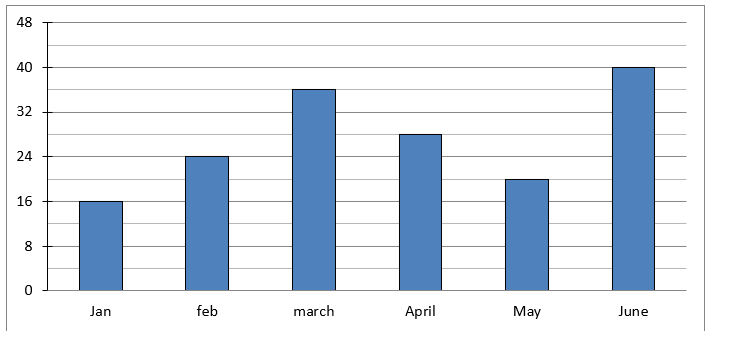Table shows the no. of questions framed by both Abhi & Satish in different month.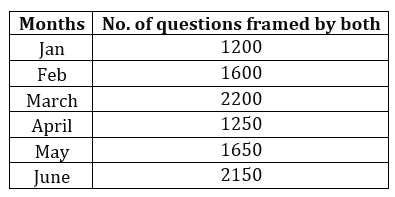Q1. No. of questions framed by another person Anurag in Jan is average of total questions framed by Abhi in Jan & questions framed by Satish in June. Find difference of total questions framed by Anurag in Jan and Abhi in feb?
(a) 481
(b) 357
(c) 383
(d) 327
(e)428

Q2. Average of questions framed by Abhi in may, june & july together is 680. Then number of questions framed by Abhi in July is approximately what percent of number of question framed by Satish in feb?
(a) 60%
(b) 65%
(c) 82%
(d) 70%
(e) 55%

Q3. If 3/4th of total no. of questions framed by Satish in March are checked by Abhi and rest remained unchecked, then find ratio of questions which remained unchecked to the average of questions framed by Abhi in Jan and Feb?
(a) 9 : 7
(b) 13 : 9
(c) 9 : 11
(d) 13 : 11
(e)11 : 9

Q4. Average number of questions framed in march and April by Abhi is approximately what percent of total question framed by both of them in May & June?
(a) 12%
(b) 23%
(c) 32%
(d) 15%
(e) 27%

Q5. What is the average number of questions framed by Satish in Jan , April and June? (a) 1280
(b)1066
(c) 1340
(d) 1156
(e) 1032

Q6. Ratio of age of Amit and his father 5 years ago is 2 : 5. If age of his mother is 20% more than the present age of his father. Then find difference of age of his father and mother after 10 years given that age of Amit 3 year hence will be 32 years?
(a) 11 years
(b) 13 years
(c) 8 years
(d) 17 years
(e) 15 years

Q7. A man invested in two different schemes A & B and investment in scheme A is 25% more than that of scheme B. Scheme A offered SI at the rate of (R – 2.5)% for two year while scheme B offered SI at the rate of (R + 5)% for three years and ratio of interest received by man from scheme A to that of scheme B is 5 : 12. Find the interest received by man, if he will invest Rs 2250 at the rate of 2R% per annum on CI for two years?
(a) 920 Rs
(b) 990 Rs
(c) 960 Rs
(d) 900 Rs
(e) 850 Rs

Q8. Ram, Veer and Virat invested Rs. 1250, (1250 + x) and (1250 + 2x) respectively in a business. After one year Divya joined them with the capital of Rs. 8x. If at the end of three years the profit of Divya is Rs. 12,000 out of total profit of Rs. 52,500, then find amount received by Virat, if he invested his initial investment’s amount at the rate of 10% per annum at CI for three years ?
(a) Rs.1929.25
(b) Rs.2029.25
(c) Rs.2129.25
(d) Rs.2229.25
(e) Rs.2329.25

Q9. Ratio between length of two trains is 1 : 2 and speed of two trains is 120 km/hr & 108 km/hr respectively and both trains running in same direction cross each other in 108 sec. If two compartments were added in smaller train then it can cross a platform of length of 12.5 times of length of one compartment in 14.04 sec, then find the time taken by longer train to cross that same platform, if five new compartments were added in to that train?
(a) 18 sec
(b) 22 sec
(c) 16 sec
(d) 20 sec
(e) 28 sec

Q10. Ratio between marked price of article P and Q is 3 : 4. Shopkeeper allowed d% discount on article P and (d + 15) % discount on article Q, due to which selling price of both articles become equal. If shopkeeper earned a profit of 8% on selling article P, and a profit of 12.5% on selling article Q and profit earned on article Q is Rs. 32 more than that of article P. Find the cost price of both articles respectively?
(a) 800 Rs. ,768 Rs.
(b) 848 Rs. ,816 Rs.
(c) 816 Rs. ,776 Rs.
(d) 824 Rs. ,792 Rs.
(e) None of these

Solutions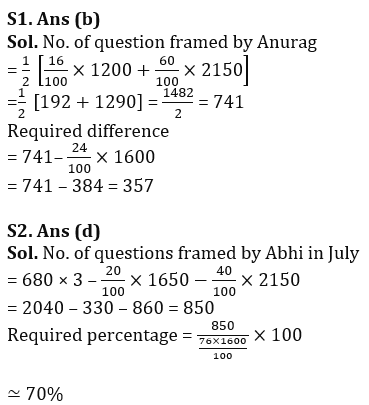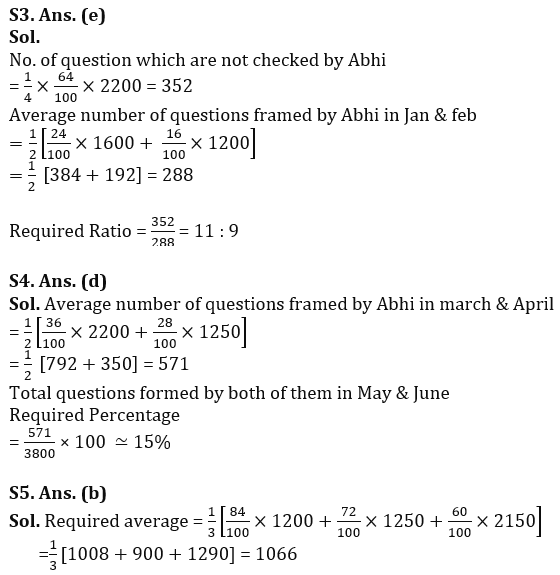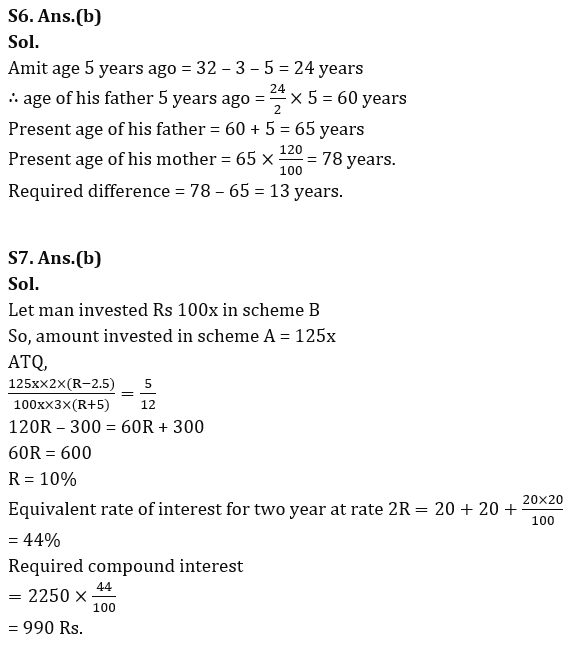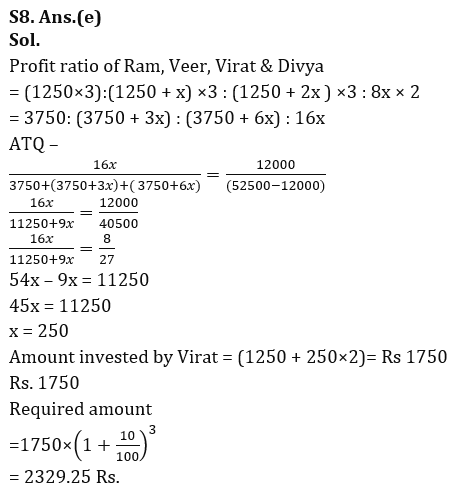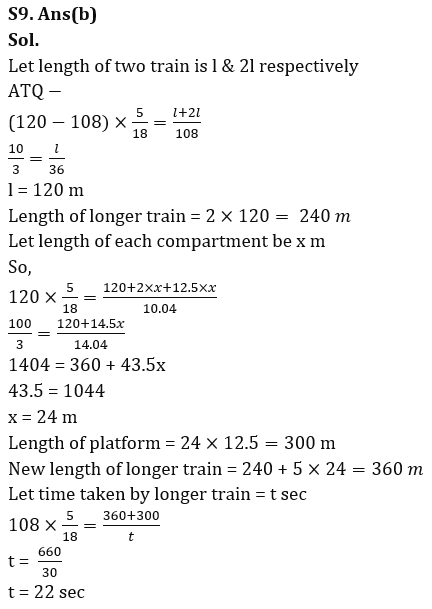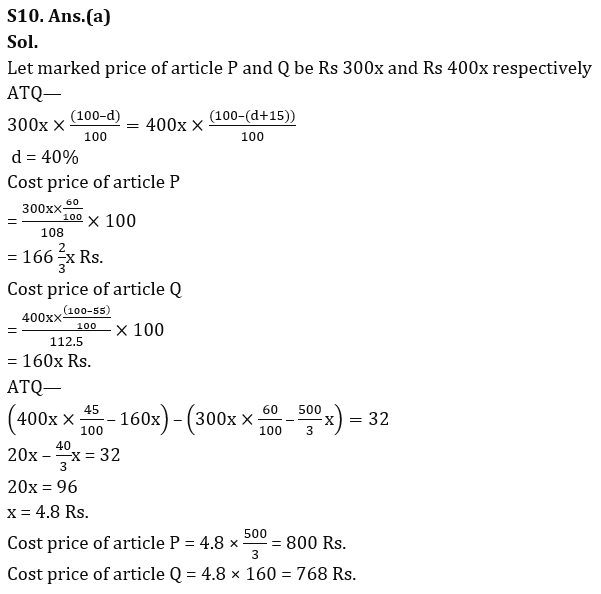## FAQs

### When will the LIC AAO Mains exam be held?

LIC AAO Mains exam will be held on 18 March

#### Congratulations!Union Budget 2023-24: Free PDF